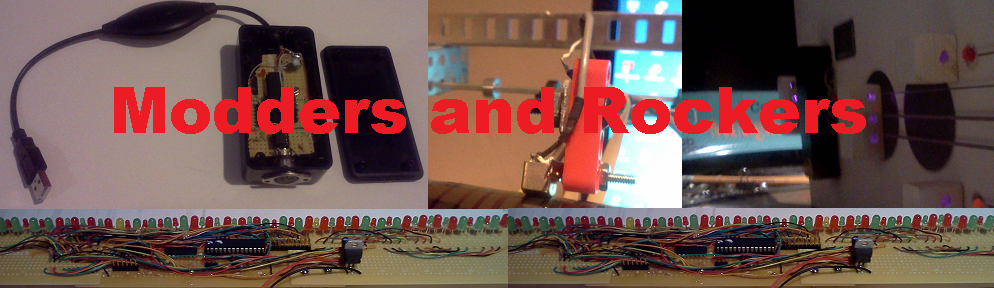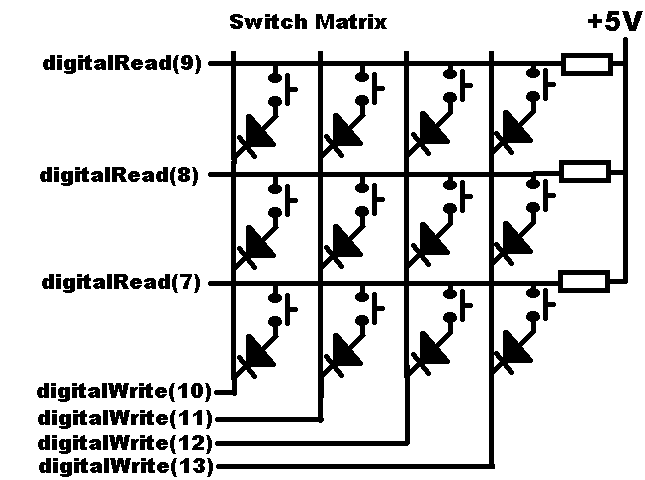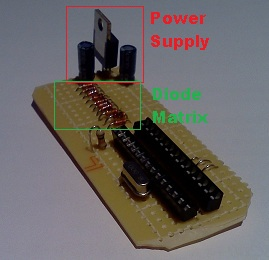Tables and Variables Switch functionThe stab table is used to determine the type of switch connected at each node in the matrix. The switches can be either Normally Open (N.O) or Normally Closed (N.C) When an N.O switch is pressed, the common row of the matrix is connected to the specific column. When the switch is released, the common row is disconnected from that column. The N.C switch is reversed. When pressed in disconnects the common row and connects it when released. The switches I used were a mixture of N.C and N.O I had left around from a previous project. The prev array holds the previous value for each switch once it has been normalized useing the stab array. The ntab array is used to determine which note is to be sent when a switch is pressed. A value of 0 means that there is no note for this switch. The mux table has the values that are used for the four outputs that are the columns of the switch matrix. The mux counter goes from 0-3. The first four values are used when the value of mux is 0. The second four values are used when the mux has the value 1 etc. Note that only one of the four is LOW at a time. The debounce array holds values used to debounce the switch contacts. When a switch is pressed, as the contacts come together they often bounce and disconnect for a moment. THey can do this several times before staying connected. The debounce array has a counter for each switch. ```// switch scanning tables // stab holds the switch type 0= N.O. 1= N.C const int stab = { 0, 0, 0, 1, // i7/s7 1, 0, 1, 0, // i8/s8 0, 0, 1, 0 }; int prev = { 1, 1, 1, 1, 1, 1, 1, 1, 1, 1, 1, 1 }; // previous value table const int ntab = { 0, 0, C5, D5, // G4+5 == C5 E5, F5, A5, G5, B5, C6, D6, E6 }; const int muxtab = { LOW, HIGH, HIGH, HIGH, HIGH, LOW, HIGH, HIGH, HIGH, HIGH, LOW, HIGH, HIGH, HIGH, HIGH, LOW }; int debounce; int mtime = 0; int mux = 0; int firstbeat= 0; int ponstate; // power on state. ``` The switches function scans the switch matrix. It uses the mux variable to select each column one at a time and read the three rows to determine which switch has changed state. The first part of the function is only used to determine what the power on state of the switches was. This code sets the ponstate variable that can be used to change functionality of the rest of the code. Things like doing debugging can use the information in the ponstate variable. ```void switches() { int i7, i8, i9; int s7, s8, s9; int midx; // this bit of code allows the initial switch debounce logic // to run it's course so we can read the initial // switch values at power up. if( mux == 3){ if( firstbeat == 0 ){ // first time firstbeat = DEBOUNCE; }else if( firstbeat <= DEBOUNCE){ --firstbeat; } if( firstbeat == 0){ // read initial switch values for(midx = 0; midx < 12; midx++){ ponstate |= prev[midx] << midx; } firstbeat = DEBOUNCE+1; // stop the processing. } } ``` The three rows are read first. The values are normalized by using the stab array. The XOR operator will flip the value if the stab entry is a 1. The midx is calculated by adding the row number to the mux variable. The current switch state is compared to the previous value stored in the prev array. If the switch state is different then the debounce array is used to see if this is a real state change. If the debounce value becomes 0 then the nChange function is called to perform the action associated with the switch. The value passed to nChange is taken from the ntab array. Using the ntab array allows the switches to be wired in any order. The debounce entry is reset to the defined DEBOUNCE value. ``` // scan the switches i9 = digitalRead(9); i8 = digitalRead(8); i7 = digitalRead(7); s9 = i9 ^ stab[mux+8]; s8 = i8 ^ stab[mux+4]; s7 = i7 ^ stab[mux+0]; midx = mux+8; if( s9 != prev[midx]){ if( debounce[midx]== 0 ){ // change in s9 nChange(s9, ntab[midx] ); prev[midx] = s9; debounce[midx] = DEBOUNCE; }else { --debounce[midx]; } }else { debounce[midx] = DEBOUNCE; } midx = mux+4; if( s8 != prev[midx]){ if( debounce[midx]== 0 ){ // change in s8 nChange(s8, ntab[midx] ); prev[midx] = s8; debounce[midx] = DEBOUNCE; }else { --debounce[midx]; } }else { debounce[midx] = DEBOUNCE; } midx = mux+0; if( s7 != prev[midx]){ if( debounce[midx]== 0 ){ // change in s7 nChange(s7, ntab[midx] ); prev[midx] = s7; debounce[midx] = DEBOUNCE; }else { --debounce[midx]; } }else { debounce[midx] = DEBOUNCE; } ``` The last part of the switches function increments the mux variable. If it is 4 then it is reset back to 0. For each of the four digital outputs that activate each of the columns of the matrix, the muxtab array is used to determine the value. ``` // update the mux ++mux; if( mux == 4){ mux = 0; } midx = mux+mux+mux+mux; digitalWrite(10, muxtab[midx]); digitalWrite(11, muxtab[midx+1]); digitalWrite(12, muxtab[midx+2]); digitalWrite(13, muxtab[midx+3]); } ```